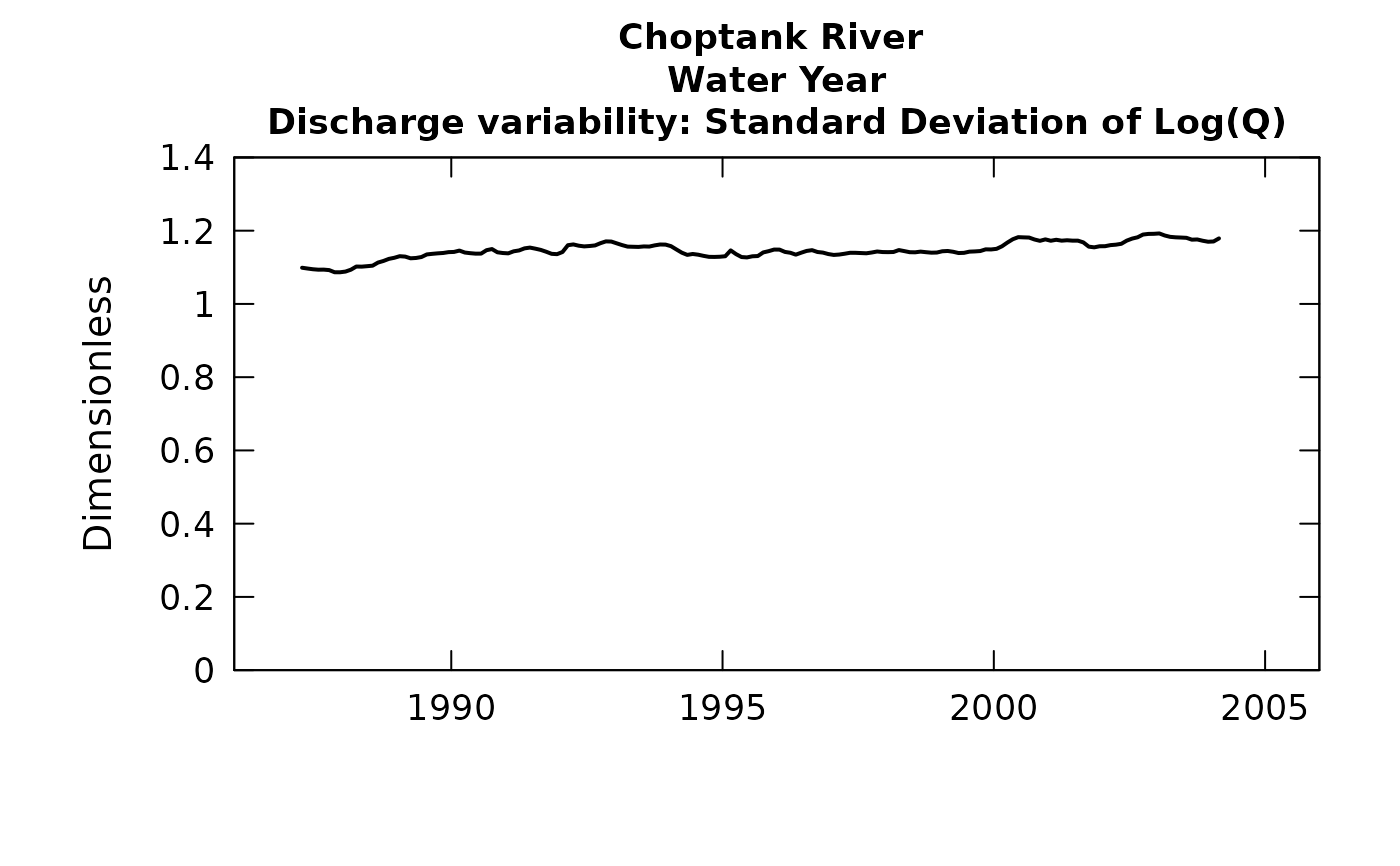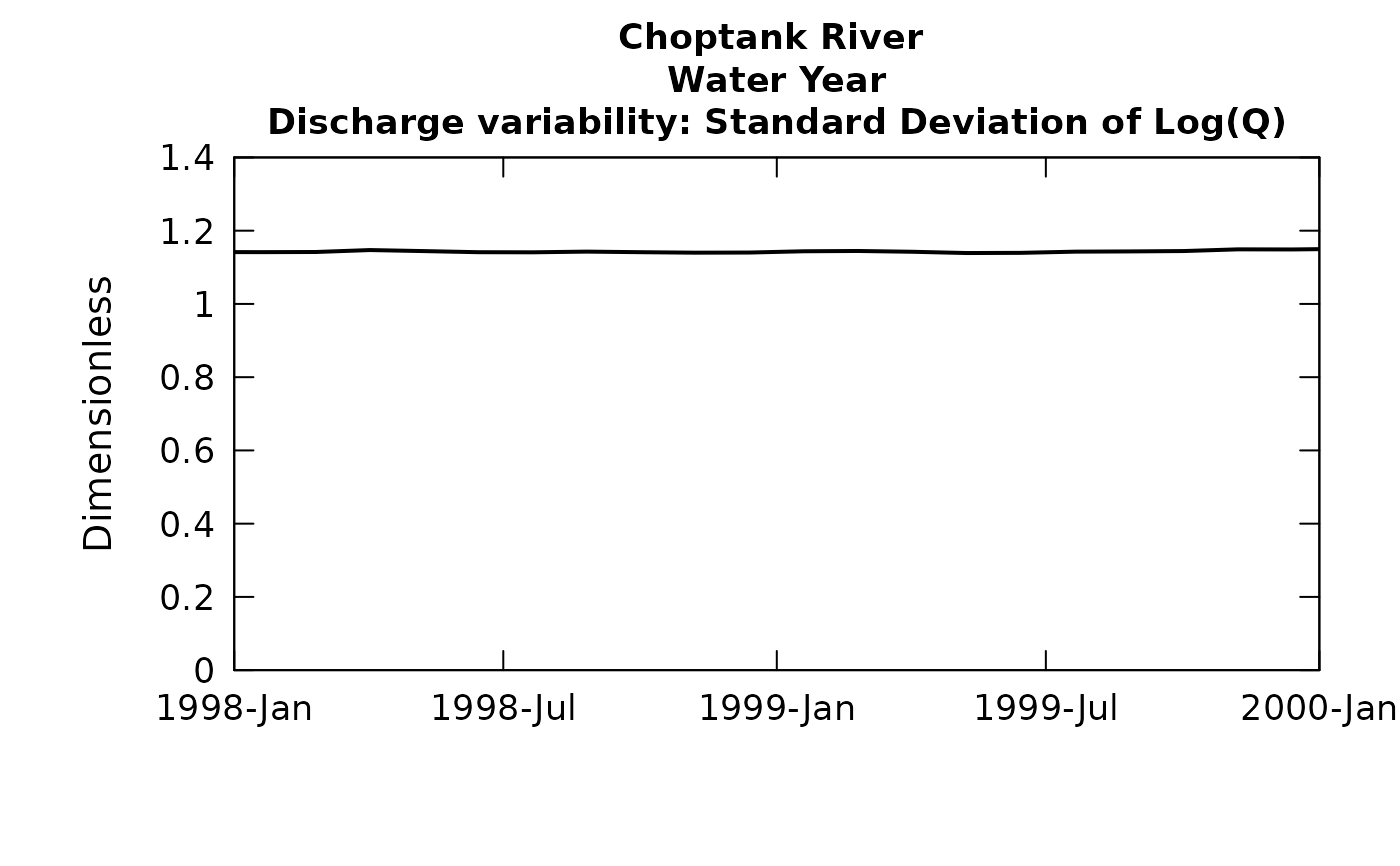Graph of the standard deviation of the log of daily discharge versus year

Although there are a lot of optional arguments to this function, most are set to a logical default.

Data come from named list, which contains a Daily dataframe with the daily flow data, and an INFO dataframe with metadata.

## Usage

plotSDLogQ(eList, yearStart = NA, yearEnd = NA, window = 15,
sdMax = NA, printTitle = TRUE, tinyPlot = FALSE, printStaName = TRUE,
printPA = TRUE, cex = 0.8, cex.main = 1.1, cex.axis = 1.1, lwd = 2,
customPar = FALSE, ...)

## Arguments

eList

named list with at least the Daily and INFO dataframes

yearStart

numeric is the calendar year of the first value to be included in graph, default is NA, which plots from the start of the period of record

yearEnd

numeric is the calendar year of the last value to be included in graph, default is NA, which plots to the end of the period of record

window

numeric which is the full width, in years, of the time window over which the standard deviation is computed, default = 15

sdMax

numeric is the maximum value to be used on the vertical axis of the graph, default is NA (which allows it to be set automatically by the data)

printTitle

logical variable if TRUE title is printed, if FALSE title is not printed (this is best for a multi-plot figure), default is TRUE

tinyPlot

logical variable if TRUE plot is designed to be small, if FALSE it is designed for page size, default is FALSE (not fully implemented yet)

printStaName

logical variable, if TRUE print the station name, if FALSE do not, default is TRUE

printPA

logical variable, if TRUE print the period of analysis information in the plot title, if FALSE leave it out, default is TRUE

cex

numerical value giving the amount by which plotting symbols should be magnified

cex.main

magnification to be used for main titles relative to the current setting of cex

cex.axis

magnification to be used for axis annotation relative to the current setting of cex

lwd

line width, a positive number, defaulting to 2

customPar

logical defaults to FALSE. If TRUE, par() should be set by user before calling this function (for example, adjusting margins with par(mar=c(5,5,5,5))). If customPar FALSE, EGRET chooses the best margins depending on tinyPlot.

...

arbitrary graphical parameters that will be passed to genericEGRETDotPlot function (see ?par for options)

selectDays, genericEGRETDotPlot

## Examples

eList <- Choptank_eList
# \donttest{
# Water year:
plotSDLogQ(eList)plotSDLogQ(eList, 1998, 2000)# }